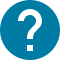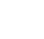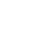学部前期課程
HOME 学部前期課程 全学自由研究ゼミナール(PEAK)(Single-variable calculus)

# 全学自由研究ゼミナール(PEAK)(Single-variable calculus)

Single-variable calculus
In this course, we study the differentiation and integration of a function of one-variable (which is also called one-variable function or single-variable function). Intuitively, differential is the slope of the tangent line of a function at a given point, and integral is the area bounded by the graph of the function and x-axis over a given interval. Both concepts need the concept “limit”, so the first part of this course will contain the definition of a function, limit of a sequence, continuous function and related topics. In the second part of this course, we study the differential of a function, the definition, calculation techniques, properties and applications. In the third part, we define the definite and indefinite integral of a function, study the fundamental theorem of calculus: the connection between differentiation and integration, and introduce the calculation techniques. Power series will also be introduced. In the end, we study how to solve some simple differential equations.

コース名

50821
CAS-PT1200S3

A1 A2

2

NO

1. Sequence, limit, continuous function 2. The derivative of a function and higher-order derivatives 3. Taylor's theorem and extreme values 4. Taylor series 5. Integral of a function 6. the fundamental theorem 7. Integration methods 8. Differential equations

Online lectures using Zoom. Homework will be given each week. Materials will be uploaded to ITC-LMS. Visit ITC-LMS for latest information.

To be determined.

Make sure you are able to spend some time on exercises/homework if you take this course. Latest information can be found on ITC-LMS.